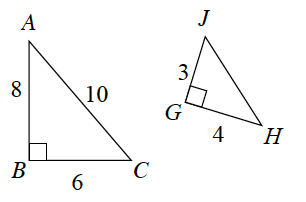Home > CC4 > Chapter 3 > Lesson 3.1.4 > Problem3-46

3-46.

$\Delta HGJ$ at right has been created from $\Delta ABC$. Why is $\Delta HGJ$ not a rigid transformation of $\Delta ABC$? Explain what properties of $\Delta ABC$ have been preserved and which have not been preserved. Homework Help ✎

Find the length of the missing side of each triangle. How are the triangles related?